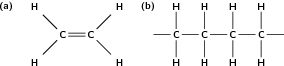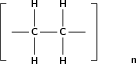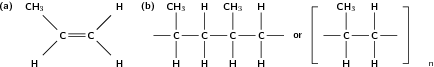Polymers

 Page 1 / 6

Organic macromolecules

As its name suggests, a macromolecule is a large molecule that forms when lots of smaller molecules are joined together. In this chapter, we will be taking a closer look at the structure and properties of different macromolecules, and at how they form.

Polymers

Some macromolecules are made up of lots of repeating structural units called monomers . To put it more simply, a monomer is like a building block. When lots of similar monomers are joined together by covalent bonds, they form a polymer . In an organic polymer , the monomers are joined by the carbon atoms of the polymer 'backbone'. A polymer can also be inorganic , in which case there may be atoms such as silicon in the place of carbon atoms. The key feature that makes a polymer different from other macromolecules, is the repetition of identical or similar monomers in the polymer chain. The examples shown below will help to make these concepts clearer.

Polymer

Polymer is a term used to describe large molecules consisting of repeating structural units, or monomers, connected by covalent chemical bonds.

1. Polyethene Chapter [link] looked at the structure of a group of hydrocarbons called the alkenes . One example is the molecule ethene . The structural formula of ethene is is shown in [link] . When lots of ethene molecules bond together, a polymer called polyethene is formed. Ethene is the monomer which, when joined to other ethene molecules, forms the polymer polyethene . Polyethene is a cheap plastic that is used to make plastic bags and bottles.(a) Ethene monomer and (b) polyethene polymer A polymer may be a chain of thousands of monomers, and so it is impossible to draw the entire polymer. Rather, the structure of a polymer can be condensed and represented as shown in [link] . The monomer is enclosed in brackets and the 'n' represents the number of ethene molecules in the polymer, where 'n' is any whole number. What this shows is that the ethene monomer is repeated an indefinite number of times in a molecule of polyethene.A simplified representation of a polyethene molecule
2. Polypropene Another example of a polymer is polypropene (fig [link] ). Polypropene is also a plastic, but is stronger than polyethene and is used to make crates, fibres and ropes. In this polymer, the monomer is the alkene called propene .(a) Propene monomer and (b) polypropene polymer

How do polymers form?

Polymers are formed through a process called polymerisation , where monomer molecules react together to form a polymer chain. Two types of polymerisation reactions are addition polymerisation and condensation polymerisation .

Polymerisation

In chemistry, polymerisation is a process of bonding monomers, or single units together through a variety of reaction mechanisms to form longer chains called polymers.

In this type of reaction, monomer molecules are added to a growing polymer chain one at a time. No small molecules are eliminated in the process. An example of this type of reaction is the formation of polyethene from ethene (fig [link] ). When molecules of ethene are joined to each other, the only thing that changes is that the double bond between the carbon atoms in each ethene monomer is replaced by a single bond so that a new carbon-carbon bond can be formed with the next monomer in the chain. In other words, the monomer is an unsaturated compound which, after an addition reaction, becomes a saturated compound.

different structural formula
different type of structural formula
Lucas
primary,secondary and tertiary
Snowy
how does the earth revolute
What is the momentum
The product of the object's mass and it's velocity
Mvula
electric field def
FREENCO
what is secondary alcohol?
Raymond
is when Carbon that is bonded with OH is also bonded to 2 carbons of the chain.
Gontse
how is ester formed
how is n ester formed
Aubrey
Alcohol reacts with a carboxylic acid
Texas
and the reaction is catalysed by sulphuric acid
Vhulenda
An ester is form when an alcohol reacts a carboxylic acid and sulphuric acid is used as a catalyst which therefore eliminates water.
Azola
an athlete with a mass of 70kg runs at a velocity of 45km . determine the athlete's momentum
Is that a velocity or something else
msawenkosi
45km/h i guess
Texas
Change to m/s
Texas
45km/h = 12.5 m/s p=mv =70×12.5 =875 kg.m/s
Thato
what are the measures of the rates of reaction
Volume Concentration Temperature Pressure Surface Area
Thato
the principle of superposition of waves
what is work
is this a group chat
Hey can y'all define newton's 2nd law
mthebzification
If a resultant force act on an object...the object will accelerate in the direction of a resultant force,the acceleration of the object is directly proportional to the net force and inversely proportional to the mass of the object
mosa
how do you calculate tension force
Bulumko
use the formula Fnet=ma if there is tension connecting two objects
Sboniso
to calculate Tension, usually calculate acceleration first Draw separate free body diagrams for each body. Apply Fnet = ma to calculate Tension
Kevin
Hi people
Paul
when a resultant force acting on an object the object will accelerate in the direction of a force at an acceleration directly proportional to the force and invesly proportional to the mass of the object.
Raymond
Hey people
Kaytee
how does temperature affect the equilibrium position
an increased temperature increases the average kinetic energy thus in turn increases the number of effective collisions........
Lwando
so...which reaction is favored between endothermic and exothermic .when temperature is increased..?
Blessing
exothermic reaction because energy is realised to the surroundings as heat and light energy ....graphical so much energy is realised as reactants to form product and because temperature is high rate of reaction is fast which means there is a successful collision
Code
INTEMENDO - INCREASE IN TEMPERATURE FAVOURS ENDOTHERMIC DETEMEXO - DECREASE IN TEMPERATURE FAVOURS EXOTHERMIC
Thato
an object will continue in a state of rest unless it is acted upon an unbalanced force
Newton's Law 1
Code
First Newton's Law
Azola
Newton's first law
Surprise
newton first law
Thinavhuyo
Newton's first law
Blessing
when pressure is increased what happen to volume
decreases
Code
care to explain?
Mpati
if pressure is applied to a pistol , the volume will decrease and particles will collide more frequently with the wall of a container .Each time they collide with the wall they exert a force on them .More collision means more force and the pressure will increase , that Boyle's Law
Code
Because the volume has decreased , the particle will collide more frequently with the wall of a container and each time they collide with the wall of a container they exert a force on them.More collision means more force so the pressure will increase , that Boyle's Law
Code
what is the difference between momentum and a change in momentum?
Change in momentum is the impulse of the object
Rachel
Momentum is a vector quantity equal to the product of the mass of the particle and its velocity
Mmola
How to name a branched molecule from right or left?
What's Free Fall
Free Fall means there is no acting force on that object.
Dingaletu
only gravitational force
Dingaletu
no external force acting on an object
Sphiwe
by only force of gravite
Sello
but gravitational force
Sphiwe
true
Lucky
a motion in which the only force acting is gravitational force
Blessing
and an object experiencing free fall is referred as a projectile
BlessingByBy MldelatteBy Rhodes